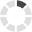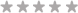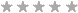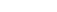### Site InformationLoading... Please wait...
• My Account

# QNT 561 Week 1 Individual Assignment Statistics Concepts and Descriptive Measures

\$10.00Quantity:## Product Description

Purpose of Assignment

The purpose of this assignment to orient students to the key concepts in statistics. This assignment will introduce students to the language of statistics. Students will also get a chance to warm-up on evaluating some basic descriptive statistics using Excel® prior to the course start.

Assignment Steps

This assignment has an Excel® dataset spreadsheet attached.  You will be required to only do one of the three datasets.

Resource: Microsoft Excel®, Statistics Concepts and Descriptive Measures Data Set

Choose one of the following datasets to complete this assignment:

• Consumer Food
• Financial
• Hospital

Answer each of the following in a total of 90 words:

• For each column, identify whether the data is qualitative or quantitative.
• Identify the level of measurement for the data in each column.
• For each column containing quantitative data:
• Evaluate the mean and median
• Interpret the mean and median in plain non-technical terms
• Use the Excel =AVERAGE function to find the mean
• Use the Excel =MEDIAN function to find the median
• For each column containing quantitative data:
• Evaluate the standard deviation and range
• Interpret the standard deviation and range in plain non-technical terms
• Use the Excel =STDEV.S function to find the standard deviation
• For range (maximum value minus the minimum value), find the maximum value using the Excel =MAX function and find the minimum value using the Excel's =MIN function

## Product Reviews

1. #### Excellent HelpPosted by on 26th Aug 2018

Helped me better understand the assignment and helped me get all points available.

## Customers also viewed

• \$15.00• \$15.00• \$10.00• \$15.00• \$15.00## Related Products

Click the button below to add the QNT 561 Week 1 Individual Assignment Statistics Concepts and Descriptive Measures to your wish list.